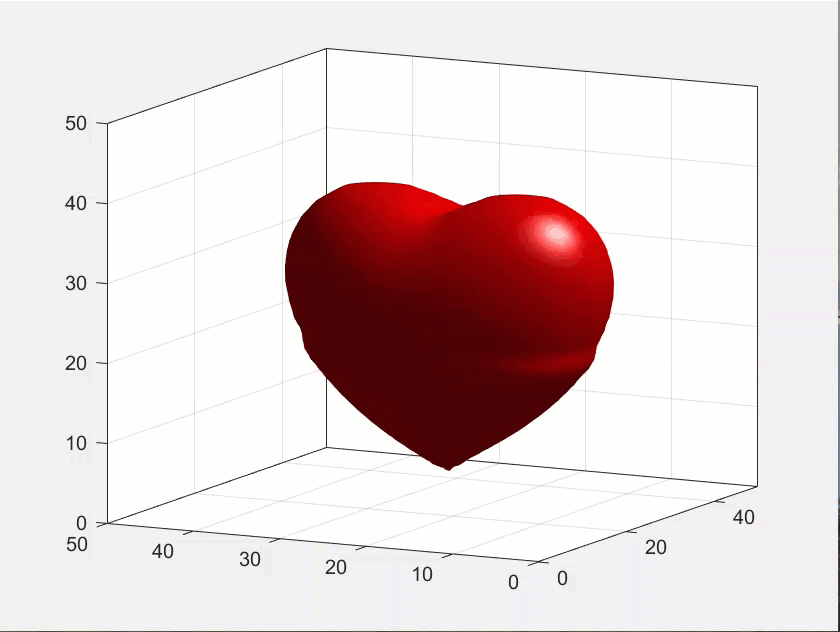## File Exchange Pick of the WeekOur best user submissions

### This is machine translation

Mouseover text to see original. Click the button below to return to the English version of the page.

# Beating (Cartoon) Heart2

Posted by Sean de Wolski,

Sean‘s pick this week is Beating (cartoon) Heart by Nathaniel Barlow.

In the spirit of celebrating Valentine’s day with heart inspired FEX submissions (, ), we add to the list with another. This is an animated cartoon heart that beats!Give it a try and let us know what you think here or leave a comment for Nathaniel.

Get the MATLAB code

Published with MATLAB® R2019a

Brett Shoelson replied on : 1 of 2
Nice Pick for Valentine's Day, Sean. I have a slight modification to suggest. Recognizing that Nathaniel's model goes through some funky phases, and looks most "heart-like" for his first calculation of t1, I changed it a bit to calculate ONLY that version, then cyclically modified the zoom factor to zoom in and out on the single image. I think it looks better...give it a try! % L = 2.5; N = 40; M = [0:(N/2) (-N/2):(-1)]'; clf; kx = 2*pi*M/L; [Kx,Ky,Kz] = meshgrid(kx,kx,kx); x = -L/2:L/N:L/2; [X,Y,Z] = meshgrid(x,x,x); Phi = 2-(2*X.^2 + Y.^2 + Z.^2 -1).^3 + 0.2*X.^2.*Z.^3 + Y.^2.*Z.^3; Phihat = fftn(Phi); gam = 0.01*(-Kx.^2-Ky.^2-Kz.^2); t1 = 0:0.05:1; t1 = [t1 fliplr(t1) t1 fliplr(t1) t1 fliplr(t1) t1 fliplr(t1) t1 fliplr(t1)]; clf; set(gcf,'color','w'); t = 1; Phi = ifftn(Phihat.*exp(gam*t1(t))); isosurface(Phi,2); lighting phong;colormap([1 0 0]); axis([0 N+N/4 0+-10 N+N/4 0 N+N/4]); view(-63,12); box off; %grid on set(gca,'XTick',[],'YTick',[],'ZTick',[],... 'xcolor','w','ycolor','w','zcolor','w') drawnow; pumpAmplitudeScaleFactor = 0.04; for ii = 1:0.5:50 zoomval = 1+pumpAmplitudeScaleFactor*sin(ii); zoom(zoomval) pause(0.05) end % Cheers! Brett
Brett Shoelson replied on : 2 of 2
(Sorry for that formatting snafu...pasting my code snippet again!)
L = 2.5; N = 40;
M = [0:(N/2) (-N/2):(-1)]';
clf; kx = 2*pi*M/L;
[Kx,Ky,Kz] = meshgrid(kx,kx,kx);
x = -L/2:L/N:L/2;
[X,Y,Z] = meshgrid(x,x,x);
Phi = 2-(2*X.^2 + Y.^2 + Z.^2 -1).^3 + 0.2*X.^2.*Z.^3 + Y.^2.*Z.^3;
Phihat = fftn(Phi);
gam = 0.01*(-Kx.^2-Ky.^2-Kz.^2);
t1 = 0:0.05:1;
t1 = [t1 fliplr(t1) t1 fliplr(t1) t1 fliplr(t1) t1 fliplr(t1) t1 fliplr(t1)];
clf;
set(gcf,'color','w');
t = 1;
Phi = ifftn(Phihat.*exp(gam*t1(t)));
isosurface(Phi,2);
lighting phong;
colormap([1 0 0]);
axis([0 N+N/4 0+-10 N+N/4 0 N+N/4]);
view(-63,12); box off;
grid on
set(gca,'XTick',[],'YTick',[],'ZTick',[],...
'xcolor','w','ycolor','w','zcolor','w')
drawnow;
pumpAmplitudeScaleFactor = 0.04;
for ii = 1:0.5:50
zoomval = 1+pumpAmplitudeScaleFactor*sin(ii);
zoom(zoomval)
pause(0.05)
end